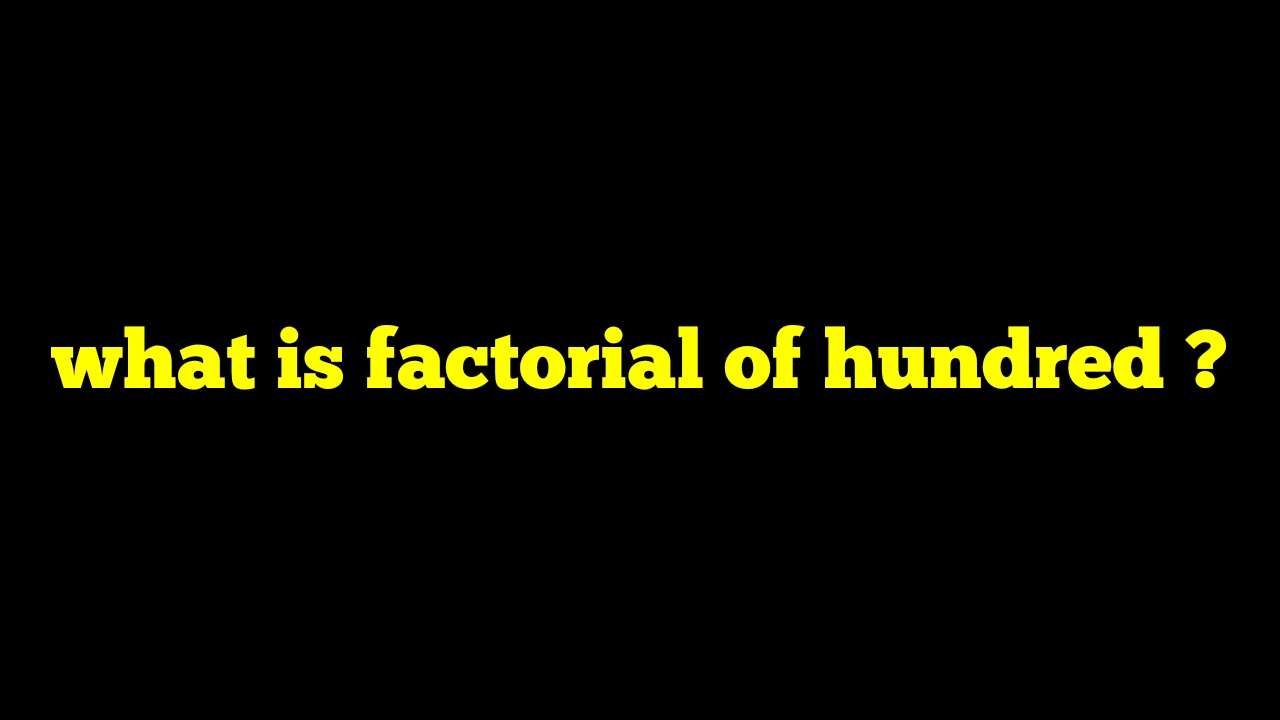If you want to knowthen it is exactly 93326215443944152681699238856266700490715968264381621468592963895217599993229915608941463976156518286253697920827223758251185210916864000000000000000000000000.

That means the approximate value of 100! Is 9.3326215443944E+157

Here, is the number of trailing zeros in the 100! Is 24 and the number of digits available in 100! Is 158. Therefore, the factorial can easily be calculated as 100! = 100*99*98*97*.........*3*2*1.In short, the factorial function is very useful in computing the number of combinations and permutations that can easily be constructed from a set of objects. Hence, the factorial of 100 is 9.33262154439441e+157

You can also apply Stirling’s approximation of factorials as well where 100! Is approximately equal to 100!≈9.32*10^157